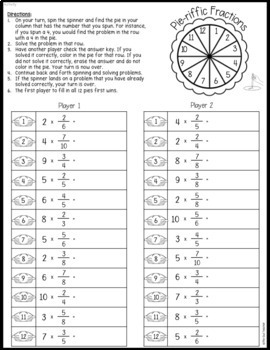# Multiplying Fractions Guided Math Workshop Lesson Plans Activities and More;
4th, Homeschool
Subjects
Resource Type
Standards
Formats Included
• PDF
•Google Apps™
Pages
225 pages
\$12.00
\$12.00
Report this resource to TPTThe Teacher-Author indicated this resource includes assets from Google Workspace (e.g. docs, slides, etc.).

### Description

Fractions can be difficult for any grade level, but it doesn't have to be. I scaffold my lessons, provide hands-on learning, and so much more so that students will truly understand multiplying fractions and that anxiety will disappear!

Save 30% off now and buy the year-long bundle!

This guided math workshop unit is complete with pre-tests, vocabulary cards, 15 days of lesson plans that are very thorough and detailed, guided math activities, interventions, and extension suggestions, math centers (such as math games), post-tests for math assessment, and much more!

You can also get a free sample of one of my math workshop units (a third-grade example) here.

Check out the preview for details!

This Unit Covers:

• Hands-on multiplication of a whole number times a fraction
• Writing fractions as a sum of unit fractions
• Finding the product of a whole number and a fraction using multiple strategies such as repeated addition, visual fractions, and the use of a number line
• Renaming fractions
• A bonus (or alternative lesson) on multiples
• Finding multiples of fractions
• Solving fraction multiplication problems
• Creating equivalent equations with a unit fraction
• Using the associative property of multiplication to help
• Multiplying mixed numbers by a fraction
• Solving word problems that involve multiplying fractions
• Analyzing line plots with fractions of one-half, one-fourth, and one-eighth
• Creating line plots with fractions of one-half, one-fourth, and one-eighth

With This Unit You'll Get:

• Pre-test and post-test
• Vocabulary cards for math word wall instruction
• Detailed lesson plans
• Anchor charts
• Differentiated math activities
• Interactive notebook pieces (INB)
• Math centers or math stations materials
• Math games
• Math worksheets for extra practice & review
• Remediation & enrichment
• Mini-assessments
• Digital & print versions
• Color & printer-friendly versions

Other Related Products You May Enjoy:

Add & Sub Fractions with Like Denominators Math Workshop (Gr 4)

Equivalent Fractions Guided Math Workshop (Gr 4)

**IMPORTANT NOTICE**

This resource is not editable. I cannot allow the resource to be editable due to the Terms of Use on the clip art used. Thank you for understanding.

Printing Notes:

This resource is printer-friendly and includes a black and white version. I would suggest printing "as image" to avoid any potential issues. Please also make sure your adobe reader (or the like program) is up-to-date.

Mistakes Happen!☜

If you see ANYTHING that needs modifying or if you have any questions, please contact me via the Q&A. I take my product creation and your satisfaction very seriously! Thanks!

Earn FREE Resources!

It's very important to me that you provide feedback so that I may improve and create products you will use and love! Please consider leaving detailed feedback. Additionally, each time you provide feedback, you earn TpT credits. These can be taken off purchases so you can get items free!

❤️Love What You See? ❤️

Join my email list here to get FREEBIES and so much more!

Follow my store here to get discounted goodies each time they're posted!

Total Pages
225 pages
Included
Teaching Duration
3 Weeks
Report this resource to TPT
Reported resources will be reviewed by our team. Report this resource to let us know if this resource violates TPT’s content guidelines.

### Standards

to see state-specific standards (only available in the US).
Make a line plot to display a data set of measurements in fractions of a unit (1/2, 1/4, 1/8). Solve problems involving addition and subtraction of fractions by using information presented in line plots. For example, from a line plot find and interpret the difference in length between the longest and shortest specimens in an insect collection.
Find all factor pairs for a whole number in the range 1-100. Recognize that a whole number is a multiple of each of its factors. Determine whether a given whole number in the range 1-100 is a multiple of a given one-digit number. Determine whether a given whole number in the range 1-100 is prime or composite.
Understand addition and subtraction of fractions as joining and separating parts referring to the same whole.
Apply and extend previous understandings of multiplication to multiply a fraction by a whole number.
Understand a fraction 𝘢/𝘣 as a multiple of 1/𝘣. For example, use a visual fraction model to represent 5/4 as the product 5 × (1/4), recording the conclusion by the equation 5/4 = 5 × (1/4).